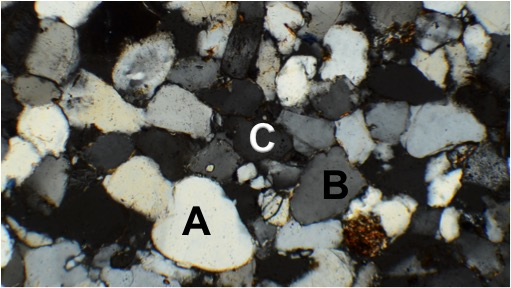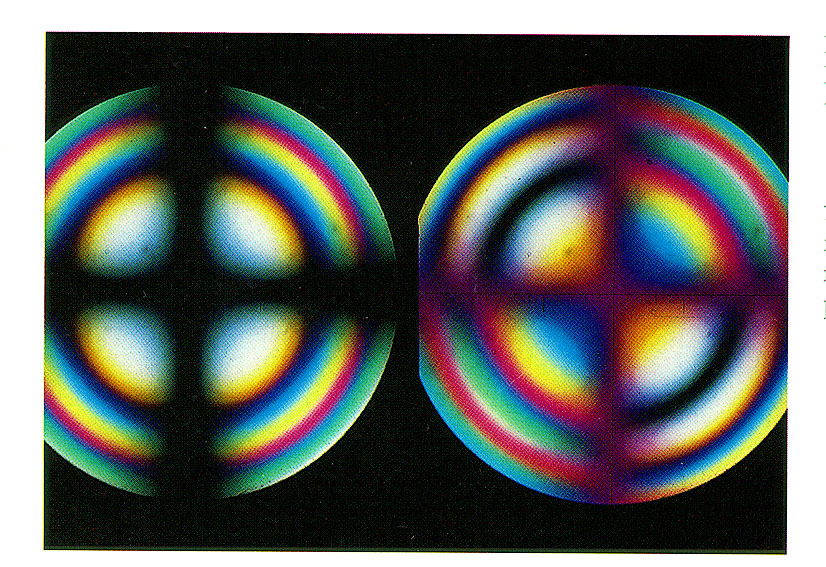Practice Quiz 4
For this exercise, please watch the first tutorial video before answering the questions. You are encouraged to use the video as much as you would like to answer the following questions. You will have an opportunity at the end to check your answers and take the quiz again for practice.
What is the order of steps you would take to obtain an interference figure?
What retardation is the best choice in obtaining an optic centered uniaxial interference figure?
In the picture below, which mineral would be the best choice to use to obtain a uniaxial optic axis interference figure?
Assume that all minerals are in their maximum illumination position.In the video below, reconstruct the uniaxial interference figure in your head as the figure rotates. When the video stops, which quadrant does it end in?
In the picture below, what is the optic sign of this material?
Assume a gypsum plate was inserted from the southeast.In the video below, what is the optic sign of the material?
Hint: The accessory plate is inserted at 0:25 seconds into the video.